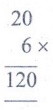Home | | Maths 4th Std | Exercise 5.4 (Money)

# Exercise 5.4 (Money)

Text Book Back Exercises Questions with Answers, Solution : 4th Maths : Term 3 Unit 5 : Money : Exercise 5.4 (Money)

Exercise 5.4

1. Priya bought 20 balloons. If the cost of one balloon is 6, then find the cost of 20 balloons.

Number of balloons = 20

Cost of 1 balloon =  6

Cost of 20 balloons = 20 x 6 =  120Answer: Cost of 20 balloons =  120

2. Chinthamani bought 28 chocolate for her birthday. If the cost of a chocolate is  7 then find the total cost of 28 chocolates?

Number of chocolates = 28

Cost of 1 chocolate =  7

Cost of 28 chocolates = 28 x 7 =  196Answer: Cost of 28 chocolates =  196

3. Ashok purchased 9 decorative papers at the cost of  450 for his town festival. Find the cost of a decorative paper?

No. of decorative papers = 9

Cost of decorative paper =  45

Cost of 1 decorative paper = 45 ÷ 9 =  5

Answer: Cost of 1 decorative paper =  5

4. Geethanjali bought 10 pencils at the cost of  70 from the supermarket. Find the cost of a pencil?

No. of pencils = 10

Cost of 10 pencils =  70

Cost of 1 pencil = 70 ÷ 10 = 7

Answer: Cost of 1 pencil =  7

5. Kuppan bought pencil and pen for  24.50 and  6.50 from his total amount  50. Find the total cost of things that he bought. Also find the balance amount?

Step: 1

Cost of pencil  =  24. 50

Cost of Pen     =  6. 50

Total               =  31.00

Step : 2

Total amount    =  50. 00

Cost of things  =  31.00

Balance            =  19. 00

Answer: Total cost of things =  31

Balance amount =  19

6. A teacher went to the science exhibition with their student. The entry fee is  250 and the teacher bought some materials for the school for  320, she had the balance amount of  330, find the amount that she had?

Entry Fee                     =  250

Cost of materials          =  320

Balance amount             =  330

Total amount she had    =  900